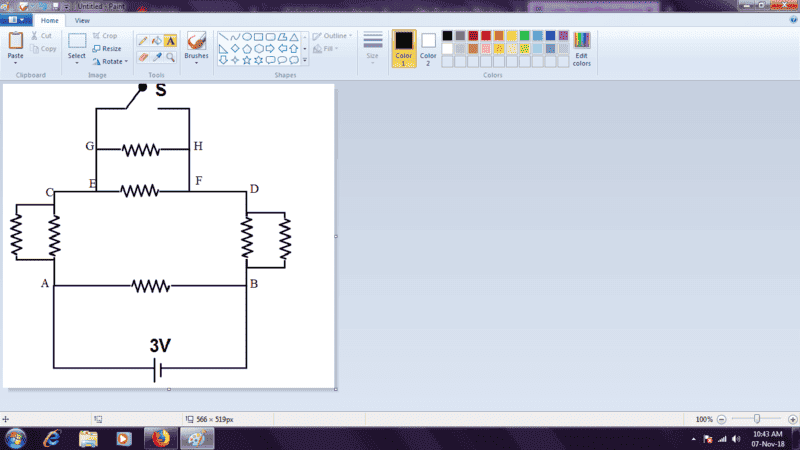# Current through circuit when switch is open

## Homework Statement

Seven equal resistors, each of resistance 2Ω are connected with a battery of 3V in a circuit shown. If i1 is the current when the switch S is open and i2 is the current when switch S is closed, find the value of i1/i2.## Homework Equations

1)Equivalent resistance formula
2)Ohm's law

## The Attempt at a Solution

If the switch is closed, it is very obvious that equivalent resistance=6/5Ω
Then, current i2=5/2 A
But the confusion occurs when we "open" the switch. My intuition says that no current should flow through ACEGHFDB. So, the required current = 3/2 A. Hence, required ratio=3:5.
But my book says that the answer is 5:6.
Please help me find out where I went wrong. Thanks.

## Answers and Replies

gneill
Mentor
If i1 is the current when the switch S is open and i2 is the current when switch S is closed...
The current where? Through what?

I mean the current through the whole circuit.

gneill
Mentor
I mean the current through the whole circuit.
There are several branches, thus several different currents. Please be specific.

Do you mean the current that the 3 V source provides?

Yes.

Delta2
Homework Helper
Gold Member
Before I say everything, what do mean when you say the switch is open/closed?? For me as it is drawn and shown in the picture, it is in open position. Is that what you mean?

In what follows I consider the switch to be "open" when it is in the shown position, and closed otherwise

When the switch is open, it is like the switch doesn't exist at all, just compute the total resistance of the whole circuit. I calculated it equal to ##\frac{6}{5}\Omega##
When the switch is closed then only the two resistances GH and EF are effectively out of the circuit as the whole current of the branch CD passes through the switch which we consider to have zero ohmic resistance. What is the total resistance of the rest circuit. I computed it as ##R_{total}=1\Omega##

@Delta2 In the figure drawn, the switch is in open position, and the source provides a current i1, and when we close the switch, the source provides a current i2.
I get what you are saying, and it is EXACTLY the thing written in my book.
Just one small query: Can you elaborate this statement of yours: "When the switch is open, it is like the switch doesn't exist at all, just compute the total resistance of the whole circuit"
I mean, if the switch is open, how can current flow in the circuit?

Delta2
Homework Helper
Gold Member
@Delta2
I mean, if the switch is open, how can current flow in the circuit?
When the switch is open the current finds its way from the battery , through the resistances at branch AC, then through the resistances GH and EF and finally through the resistances at branch DB. I repeat again the open switch is like it doesn't exist.

On the contrary the closed switch short circuits the resistances GH and EF and it is like the resistances GH and EF don't exist, instead it exists a short circuit from C to D through which the current pass through.

I have to admit that this is a bit tricky . We are used to think that if there is an open switch somewhere in a circuit then this certainly affects the flow of current, however this is not the case here, because the switch is placed in a tricky way.

@Delta² Thanks, I have got it now.

•Delta2